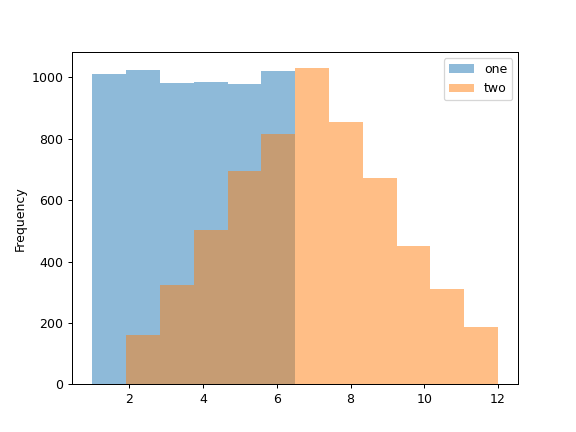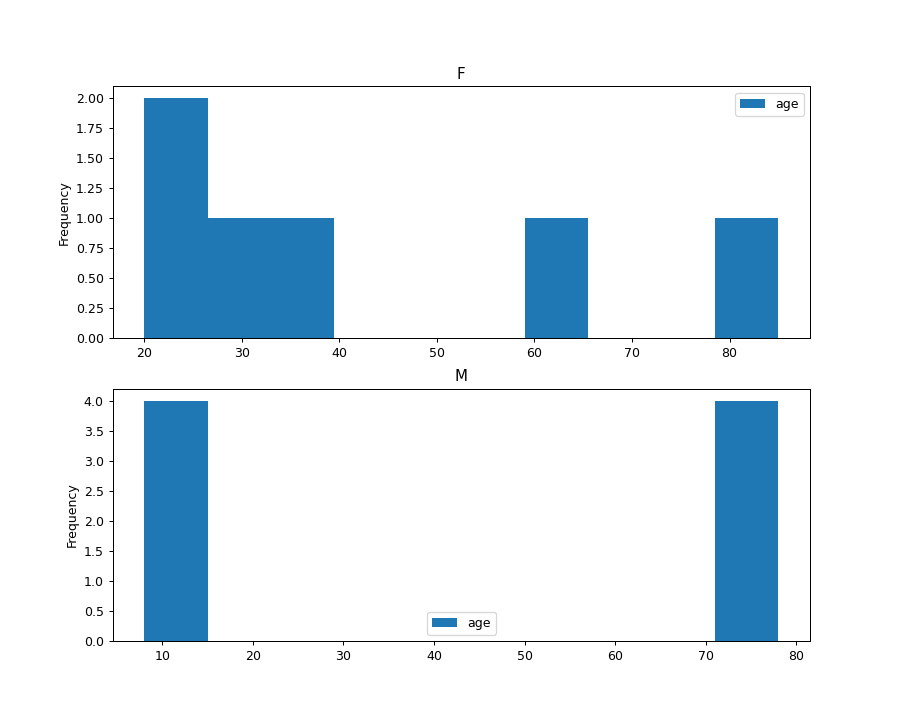# pandas.Series.plot.hist¶

`Series.plot.``hist`(by=None, bins=10, **kwargs)[source]

Draw one histogram of the DataFrame’s columns.

A histogram is a representation of the distribution of data. This function groups the values of all given Series in the DataFrame into bins and draws all bins in one `matplotlib.axes.Axes`. This is useful when the DataFrame’s Series are in a similar scale.

Parameters
bystr or sequence, optional

Column in the DataFrame to group by.

Changed in version 1.4.0: Previously, by is silently ignore and makes no groupings

binsint, default 10

Number of histogram bins to be used.

**kwargs

Additional keyword arguments are documented in `DataFrame.plot()`.

Returns
class:matplotlib.AxesSubplot

Return a histogram plot.

`DataFrame.hist`

Draw histograms per DataFrame’s Series.

`Series.hist`

Draw a histogram with Series’ data.

Examples

When we roll a die 6000 times, we expect to get each value around 1000 times. But when we roll two dice and sum the result, the distribution is going to be quite different. A histogram illustrates those distributions.

```>>> df = pd.DataFrame(
...     np.random.randint(1, 7, 6000),
...     columns = ['one'])
>>> df['two'] = df['one'] + np.random.randint(1, 7, 6000)
>>> ax = df.plot.hist(bins=12, alpha=0.5)
```A grouped histogram can be generated by providing the parameter by (which can be a column name, or a list of column names):

```>>> age_list = [8, 10, 12, 14, 72, 74, 76, 78, 20, 25, 30, 35, 60, 85]
>>> df = pd.DataFrame({"gender": list("MMMMMMMMFFFFFF"), "age": age_list})
>>> ax = df.plot.hist(column=["age"], by="gender", figsize=(10, 8))
```